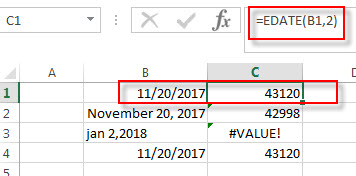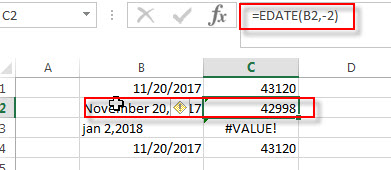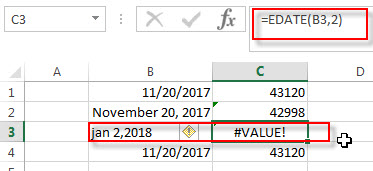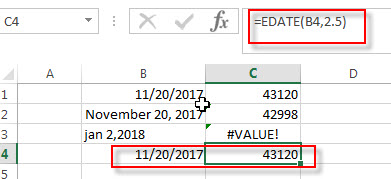# Excel EDATE Function

This post will guide you how to use Excel EDATE function with syntax and examples in Microsoft excel.

## Description

The Excel EDATE function returns the serial number that represents the date that is a specified number of months before or after a specified date. You can use the EDATE function to calculate the expiration dates or maturity dates or due dates that fall on the same day of the month as the date of issue. or you can add a specified number of months to a date.

The EDATE function is a build-in function in Microsoft Excel and it is categorized as a  DATE and TIME Function.

The EDATE function is available in Excel 2016, Excel 2013, Excel 2010, Excel 2007, Excel 2003, Excel XP, Excel 2000, Excel 2011 for Mac.

## Syntax

The syntax of the EDATE function is as below:

`=EDATE (start_date, months)`

Where the EDATE function arguments are:

• Start_date -This is a required argument. Adate that represents the start date.
• months – This is a required argument. the number of months before or after start_date. if you provide a positive number for months, it represents the future date; if it is a negative number, then it represents a past date.

Notes:

• Microsoft Excel stores dates as a serial number, it indicated the number of days since January 1,1900. and the date January 1,1900 is serial number 1.
• if start_date is not a valid date, the EDATE function will return the #VALUE! error.
• if months is not an integer, it is truncated.

## Examples

The below examples will show you how to use Excel EDATE Function to return the serial number.
1# set a positive number for months argument in the EDATE function

`=EDATE(B1,2)`2# set a negative number for months argument in the EDATE function, using the following formula:

`=EDATE(B2,-2)`3# set a invalid date for start_date argument in the EDATE function, type the following formula:

`=EDATE(B3,2)`4# set a value that is not an integer for months arguments, using the following formula:

`=EDATE(B4,2)`### More Excel EDATE Function Examples

• Conditional Formatting date with red Amber or Green
How to highlight the date with red if the cell dates is past now(). How to highlight the date with amber if the cell date is past now but within the next 6 months from now(). How to highlight the date with green if the cell date is more than 6 months from now…